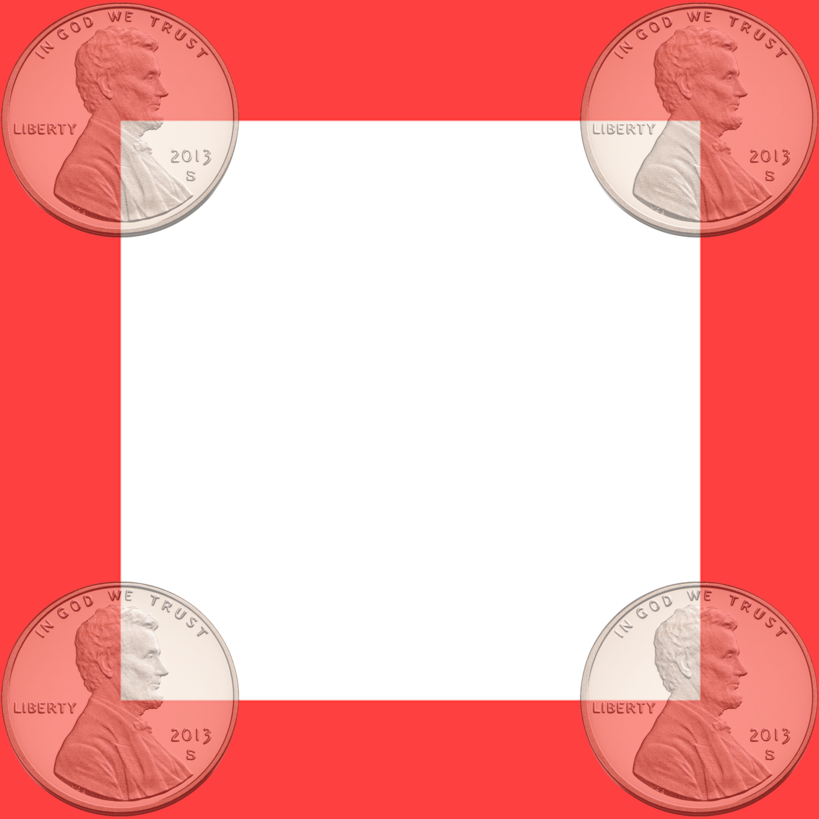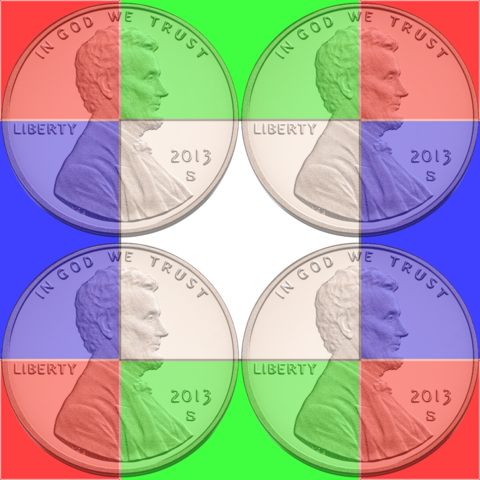# atoponce/more-coin-bits

Extracting 2 bits with every coin toss, with nothing more than one coin and a sheet of paper.Fetching latest commit…
Cannot retrieve the latest commit at this time.
Type Name Latest commit message Commit time
Failed to load latest commit information.2-bits3-bitsREADME.md

# Getting More Outcomes (bits) per Coin Toss

Instead of getting only two possibilities from a single coin toss, it is possible to get 4 outcomes (2 bits) and even 8 outcomes (3 bits) from that single toss. However, it requires a sheet of paper with a grid printed on it, and that is what this repository is all about.

## Introduction

Traditionally, only 1-bit of randomness can be extracted per coin flip- heads or tails. This design uses paper with a carefully placed grid, based on each coin diameter, to extract a uniformly distributed 2-bits from each coin toss.

## Theory

18th century mathematician Gearges-Louis Leclerc, Comte de Buffon played a common game tossing a coin onto a tile floor, betting on whether the coin landed squarely in the tile, or if the coin crossed the grout between tile squares.

He proposed calculating the probability `P` that a coin would cross the grout if you knew the coin's diameter `d` and the length of a tile edge `l`.

The probability is:

``````P = (l-d)^2/l^2
``````

By applying this formula to a printed grid on a sheet of paper, we can extract more randomness out of a single coin toss.

### Extracting 2-bits

In this case, we want to know when the probability is exactly 50%. Thus:

``````1/2 = (l-d)^2/l^2
``````

If you know the coin's diameter, you can solve for the tile edge length:

``````1/2 = (l-d)^/l^2
l^2 = 2*(l-d)^2
l^2 = 2*l^2-4*l*d+2*d^2
0 = l^2-4*l*d+2*d^2
``````

Applying the quadratic formula as a solution to our quadratic equation, we can solve for `l` and simplify, yielding:

``````l = d*(2+Sqrt(2)), l = d*(2-Sqrt((2))
``````

We are only interested in the continuous distribution of probabilities in the range of [0,1], as such, we only care about `l = d*(2+Sqrt(2))` as our solution to finding the grid edge length, when we know the coin's diameter.

#### Visual Construction

When looking at where the coin's center lands on the grid, if it lands in the white area in the following image, the edges of the coin will not cross a grid edge. However, if the center of the coin lands anywhere within the red area, then it will.Of course, as a sanity check, the area of the red should equal the area of the white. However, we have already proved that above.

### Extracting 3 bits

It is possible to extract 3 bits from a coin flip by applying the above procedure into two dimensions. Rather than testing whether or not the coin crosses any grid edge, we identify if the coin:

1. Does not cross a grid edge.
2. Crosses only any x-axis grid edge.
3. Crosses only any y-axis grid edge.
4. CRosses both the x/y axes (lands in a corner).

Using the quadratic formula that we applied above to the 1-dimensional case (whether or not the coin crossed a grid edge), can be applied on the 2-dimensional case. The equation then becomes:

``````1/4 = (l-d)^2/l^2
``````

If you know the coin's diameter, you can solve for the tile edge length:

``````1/4 = (l-d)^/l^2
l^2 = 4*(l-d)^2
l^2 = 4*(l^2-2*d*l+d^2)
l^2 = 4*l^2-8*l*d+4*d^2
0 = 3*l^2-8*l*d+2*d^2
``````

Applying the quadratic formula as a solution to our quadratic equation, we can solve for `l` and simplify, yielding:

``````l = 2*d, l = 2/3*d
``````

The solution `l = 2*d` is what we're interested in. This project provides both the 2-bits per flip and 3-bits per flip grids.

#### Visual Construction

When looking again at the coin's center, and where it lands in the grid, if it lands in the white area in the following image, the edges of the coin will not cross a grid edge. However, if the center lands anywhere in a blue area, then it will only cross a y-axis grid edge. If the center lands anywhere in a green area, then it will only cross an x-axis grid edge. Of course, if the center of the coin lands anywhere in a red area, then the coin will cross both the x and y axes by landing in a corner.As a sanity check, the area of the white equals the area of the green which also equals the area of the blue as well as the area of the red.

Some other ideas could extract a 4th bit via colored grids. This approach, and others are not currently investigated here.

## Execution

Print off the PDF appropriate for your coin and whether or not you are trying to extract 2 or 3 bits from the coin toss. Follow examples below for a demonstration on how to record the data.

If the coin lands outside of the paper, you can only record one bit, the face result, but not whether or not it crossed a grid edge, due to ambiguity.

### 2-bits

Record "tails" as least significant bit "0" and "heads" as least significant bit "1". Record if it does not cross a grid edge ("free") as most significant bit "0", and if it does cross a grid edge as most significant bit "1".

#### Example

Toss Edge Face Interpretation
1 0 1 free, heads
2 1 1 on edge, haids
3 0 0 free, tails
4 0 1 free, heads
5 1 0 on edge, tails
6 0 0 free, tails

Twelve bits have been recorded as: `01, 11, 00, 01, 10, & 00`

### 3-bits

Record "tails" as least significant bit "0" and "heads" as least significant bit "1". Record not crossing an axis ("free") as "00", crossing the x-axis as "01", crossing the y-axis as "10", and both axes (in the corner) crossing as "11".

#### Example

Toss Axis Face Interpretation
1 01 1 x-axis, heads
2 11 0 corner, tails
3 00 0 free, tails
4 11 1 corner, heads
5 11 0 corner, tails
6 10 1 y-axis, heads
7 00 1 free, heads
8 01 0 x-axis, tails
9 10 1 y-axis, heads

Twenty-seven bits have been recorded as: `011, 110, 000, 111, 110, 101, 001, 010, & 101`.

You can’t perform that action at this time.